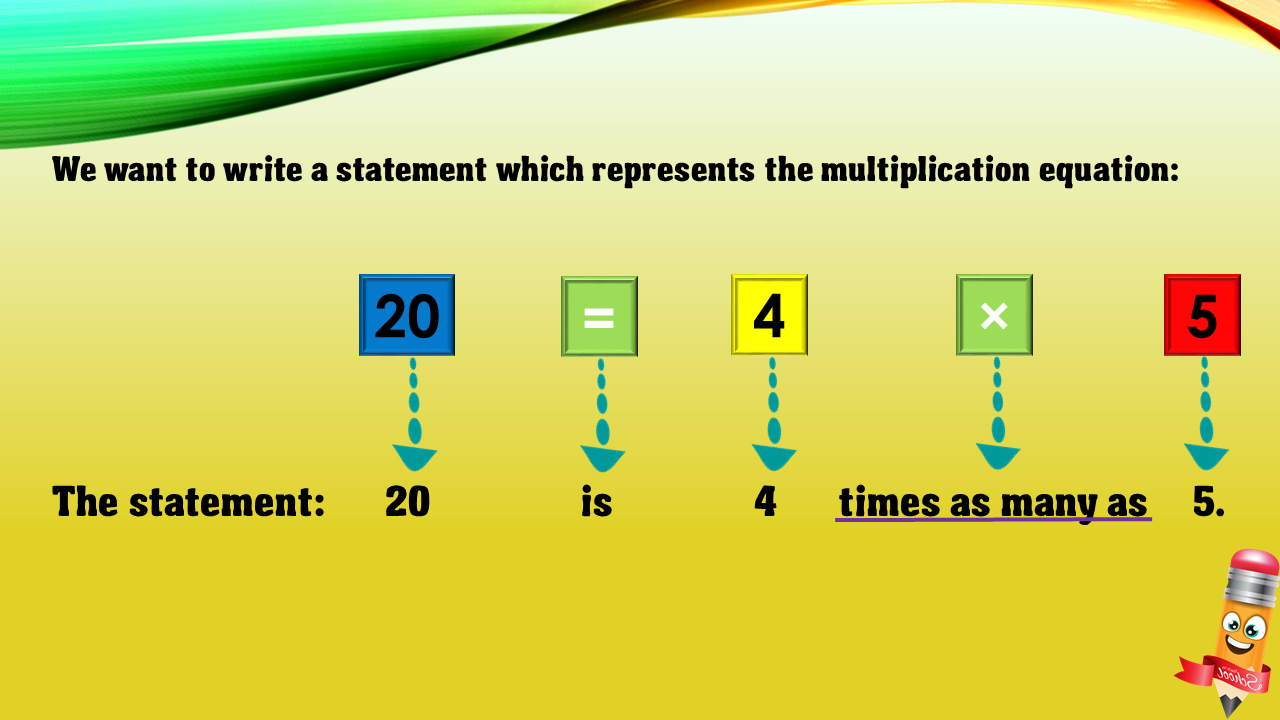1
visibility

Choose one statement which represents the equation: 20 = 4×5 ?

• A

20 times as many as 4 is 5.

• B

20 is 4 more than 5.

• C

4 is 5 less than 20.

• D

20 is 4 times as many as 5.